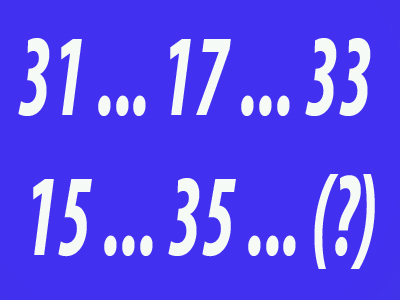Work out which number will complete the series.

# VR - Number Series Using Alternating Terms

Number Series questions are very similar to the Letter Series questions we look at in another article, only we are dealing with numbers rather than with letters.

Arithmetic and Geometric Progression, along with Alternating Terms and Geometric Progression, and Negative Arithmetic Progression, are three other ways to work out the next number in a series. We'll look at them in the next three articles. They are all a test of mathematic ability, as well as logic and reason.

In this particular article, we look at number series which are formed through the use of alternating terms.

## How Are These Kind Of Questions Posed in The Exam?

Whatever the method used to create them, Number Series questions are put to candidates in the same way. Children are shown an incomplete series of numbers and asked to add the missing number.

I’m sure we all remember similar questions from our maths lessons at school. Even so, to refresh our memories, let’s show you some examples:

Example Question One

Use the following series of numbers to determine what should come next.

 50 40 45 42 40 44 (?)

Immediately you should be checking that your child has scanned across the first three numbers to see that they are all increasing or decreasing. They aren’t, so common sense suggests that we need to work through alternate numbers as that should give us a far more straightforward view.

We are trying to find the seventh number so we need to look at the first, third and fifth in the sequence - in this case the second, fourth and sixth figures should have absolutely no bearing on the answer required. So, that gives us -

 50 … 45 … 40 …

The series is now much simpler – taking five off each number. The answer will therefore be 35. We have ignored half of the question and don’t need to know anything about it – there’s plenty more to do in the paper without doing what the other children will be working their way through!

Let's try another one in a near-identical vein:

Example Question Two

Use the following series of numbers to determine what should come next.

 31 17 33 15 35 (?)

Given that the sequence goes up and down, all we need to do is concentrate on the second, fourth and sixth terms of the sequence. Taking out the first, third and fifth terms gives us this -

 … 17 … 15 …

The answer will therefore be 13.

Example Question Three

Use the following series of numbers to determine what should come next.

 32 17 16 23 8 29

This question proves that you need to check out more than just the first three numbers to determine whether they all increase or decrease. The first three decrease, the fourth then increases. We must therefore assume that we must deal with alternating numbers.

We need the seventh number in the sequence so let’s look at the first, third and fifth:

 32 … 16 … 8 …

This is a geometric sequence where the number halves each time. If we divide the third number by two then we end up with our answer: 4.

Let's try a second question which does the same thing but uses even, rather than odd numbered terms in the progression.

Example Question Four

Use the following series of numbers to determine what should come next.

 7 3 6 6 5 12 4 (?)

Straight away we should look at alternate terms as the sequence doesn't go up or down but fluctuates. The eighth term is the one we want so let's separate out the even numbered terms:

 … 3 … 6 … 12 …

This shows that the even numbered terms are doubling, and the eighth term should be 24.

When we separate out the two combined series we find life so much easier, but this is not a technique widely taught. Make sure your child is able to spot when it’s advantageous to do so.

## Sample Tests

So that’s Number Series Using Alternating Terms. There is a little more to tell you about these, which we’ll do in the next article. However, if you fancy a break from reading, and want to try some Number Series quizzes, then you’ll be pleased to hear that we have four of them on the Education Quizzes website.

They can be found in our Eleven Plus Verbal reasoning section, or you can follow these links:

Number Series 1

Number Series 2

Number Series 3

Number Series 4

After that you may want to learn some more techniques that might be employed in the exam. See you in the next article!

We use cookies to make your experience of our website better.

To comply with the new e-Privacy directive, we need to ask for your consent -